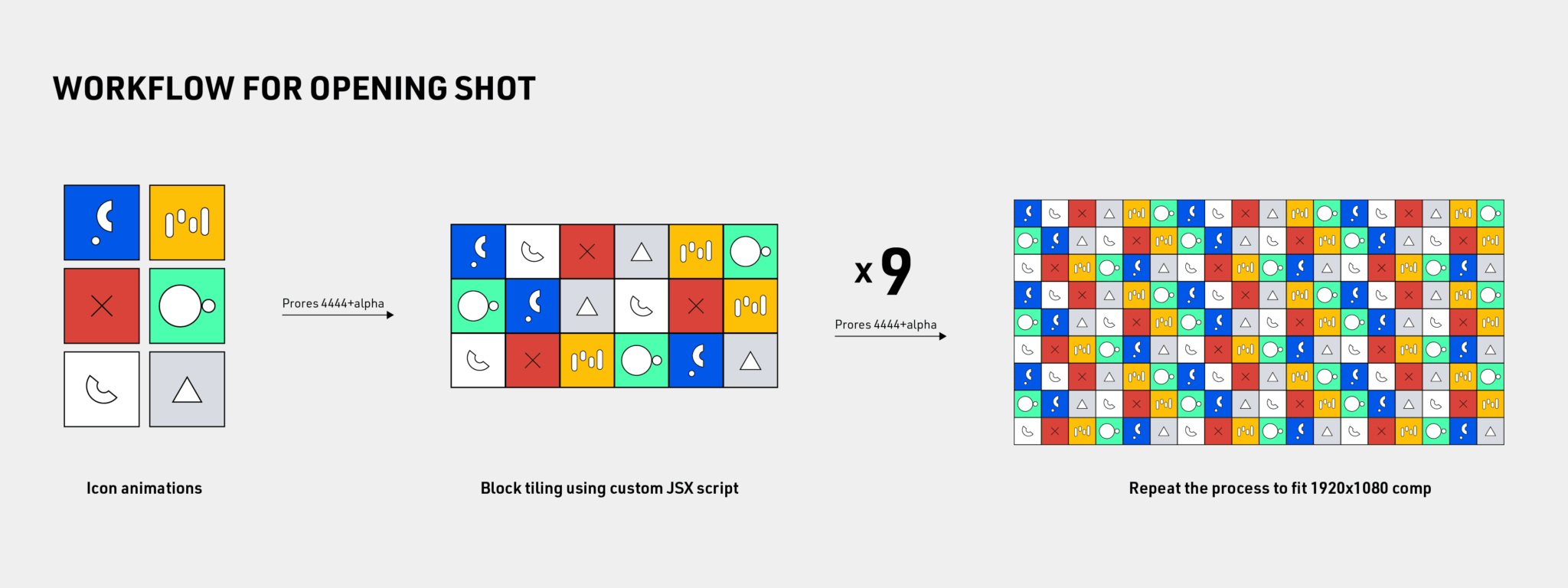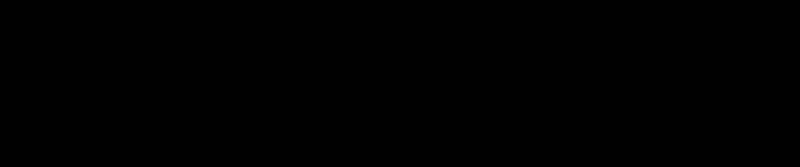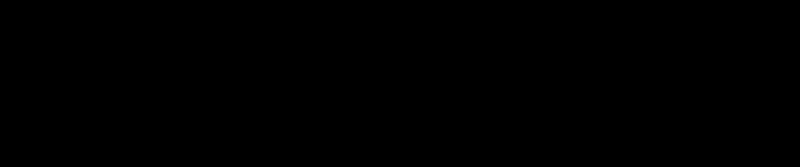Reset

This show opening was done for Vox’s Reset podcast, hosted by Arielle Duhaime-Ross, which covers why and how technology is changing everything. Inspired by Ordinary Folk’s Age of No Code animation, abstract forms and shapes were utilized as the tools for creating a simple yet compelling visual language.

I was responsible for the creative direction, project management, complex animations, and technical development such as writing a custom JSX scriptUI to automate the brick tessellation of hundreds of icons in the opening shot.

Tools and Software used: After Effects, Javascript scripting

# Design

## Visual language

Designed by Anna Yang.

# Development## Workflow

The complexity of the opening shot was mostly the tediousness of arranging hundreds of blocks or sprites into rows. Instead of doing things manually, I developed an AE JSX scriptUI that allows users to tile selected layers into brick tessellation with user-specified horizontal and vertical spacing and not have them non-repeating from the previous layer, in a single click.

However, the script is only a component of production, what matters is how the script was used. To tackle the complexity of the opening shot and eliminate the menial task of tiling layers together, here is the workflow I developed

1. Animate a series of icons of animations that spans 8 seconds.
2. Generate 9 tile tessellation combinations using the custom written script.
3. To sequential animate in the icons, a distanced-base Time Remapping expression was added to each icon layer.
4. These blocks are then rendered with alpha, and used to assemble into a full-HD composition.

## Brick tessellation script

Below are the demonstration of the aforementioned script being used, a visual diagram of how it works, and the main algorithm.

### Algorithm

```				```
// Setup
var myComp = app.project.activeItem;
var myLayers = myComp.selectedLayers;
// ----------------------------------------

// Utilities import
function getRandNum(max) {
var x = Math.floor(Math.random() * max);
return x;
};

function arraySource(array) {
var arrSrc = [];
for (i = 0; i < array.length; i++) {
arrSrc.push(array[i].source.name);
}
return arrSrc;
}

function arrayCompress(array) {
// generate array of unique names
var str = array.sort().join('\r') + '\r';
str = str.replace(/([^\r]+\r)(\1)+/g, '\$1');
str = str.replace(/\r\$/, '');
return str.split('\r');
}

// ----------------------------------------

function sortUniqueArray(array) {
var seq = [];
var str = arraySource(array);
var i = array.length;
while (i > 0) {
var groups = arrayCompress(str).length;
var randIndex = getRandNum(array.length); // random element index
var randElem = array[randIndex]; // element selection

// check for non-repeating
if (seq.length > 0) {
while (groups != 1 && seq[seq.length - 1].source.name == randElem.source.name) {
var randIndex = getRandNum(array.length);
var randElem = array[randIndex];
}
}

// append & remove
seq.push(randElem);
array.splice(randIndex, 1);

i--;
}
return seq;
}

function tileByDistance(layers, hSpace, vSpace, threshold) {
app.beginUndoGroup("tileLayers");
// Set start position
var currentTime = app.project.activeItem.time;
// var startPos = [0, 0];
var startPos = [layers.sourceRectAtTime(currentTime, false).width * layers.transform.scale.value, layers.sourceRectAtTime(currentTime, false).height * layers.transform.scale.value] / 100 / 2;
layers.transform.position.setValue(startPos);

// Tile layers
for (i = 1; i < layers.length; i++) {
myLayer = layers[i];
previousLayer = layers[i - 1];

s1 = myLayer.sourceRectAtTime(currentTime, false).width * myLayer.transform.scale.value / 100 / 2;
s2 = previousLayer.sourceRectAtTime(currentTime, false).width * previousLayer.transform.scale.value / 100 / 2;
xPos = previousLayer.transform.position.value + s1 + hSpace + s2;
yPos = previousLayer.transform.position.value;

if (xPos > threshold) {
xPos = getRandNum(s1);
yPos += vSpace;
}

myLayer.transform.position.setValue([xPos, yPos]);
}
}
// ----------------------------------------

// Main
if (myLayers != 0) {
var mySeq = sortUniqueArray(myLayers);
tileByDistance(mySeq, 0, 0, 1920);
} else {
alert("No layers are selected")
}
```
```

# Animation

## Art-directing the sequential animationLinear left to right appearanceRow by row appearance

Also, for the opening shot, instead of having the icon animation appearing in linear fashion from left to right (left image), the next challenge was to stylize the sequence in which the blocks appeared as if they were being typed row by row (right image).

Using a simple distanced-based Time Remapping expression technique with a control null layer is insufficient because it behaves as an omnidirectional effector and we wanted the blocks to appear row by row. Using the index number of layers to stagger the animation will be menial if we wanted to change the design quickly with the script I developed, hence the solution is still a distanced-based one.

To solve this, all I had to do is to measure the length of X and Y coordinates separately, and compound the time remap offset using the individual distance. Below is a demonstration of how the layers appear row by row and also the opacity expression that perform this function:

```				```
//user variables
tgt = thisComp.layer("control");
delayX = 1;
delayY = 2;
distanceX = 1920;
distanceY = 400;

// main
d1 = length(tgt.toWorld(tgt.transform.anchorPoint),transform.position)
d2 = length(tgt.toWorld(tgt.transform.anchorPoint),transform.position)
offsetx = linear(d1,0,distanceX,0,delayX);
offsety = linear(d2,0,distanceY,0,delayY);

//main
time-offsetx-offsety
```
```

## Abstract code type-on

For this shot, it was done by making animation rig that generate unique combinations of layers automatically using expressions and Master Properties. How this works is that:

1.  A unique number combination is generated using a text layer and expression (as shown below).
2. Random shape layers in a pre-composition are then accessed through the Time Remap using the values from number combination as frame number.
3. The setup is then rigged with Master Properties to create more variants by modifying the seed value.

### Generate unique number combination

```				```
var nums = [1,2,3,4,5,6,7,8,9,10],//all numbers to be randomized
ranNums = [],
i = nums.length,
j = 0;

while (i--) {
j = Math.floor(Math.random() * (i+1));
ranNums.push(nums[j]);
nums.splice(j,1);
}
ranNums
```
```

# Credits

Creative Direction: Desmond Du

Technical Direction: Desmond Du

Art Direction & Design: Anna Yang

Animators: Desmond Du, Sabrina Guyton